You have no items in your shopping cart.
Search

# Irrigation System Required Total Flow Rate

Use this form to calculate the system capacity (flow rate) required to meet the demands of a field.

## System Pumping Requirements Calculator

Net Application Required Per Week:
Total Area:
Total Hours Available to Operate per Day:
hrs
Total Days Available to Operate per Week:
days per week
Irrigation Efficiency:
%
Total Flow Required to Operate System:

### The Equation

This calculator uses this formula to determine the system pumping requirements of a field.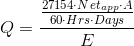Where: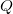= Total flow rate required to operate irrigation system (gpm)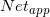= The net application required per week (in)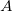= Total area to be irrigated (acres)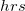= Total hours available to operate per day (hrs)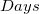= Total days available to operate each week (days)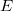= Efficiency of the irrigation system (as a deciaml)

Reference: Washington State University